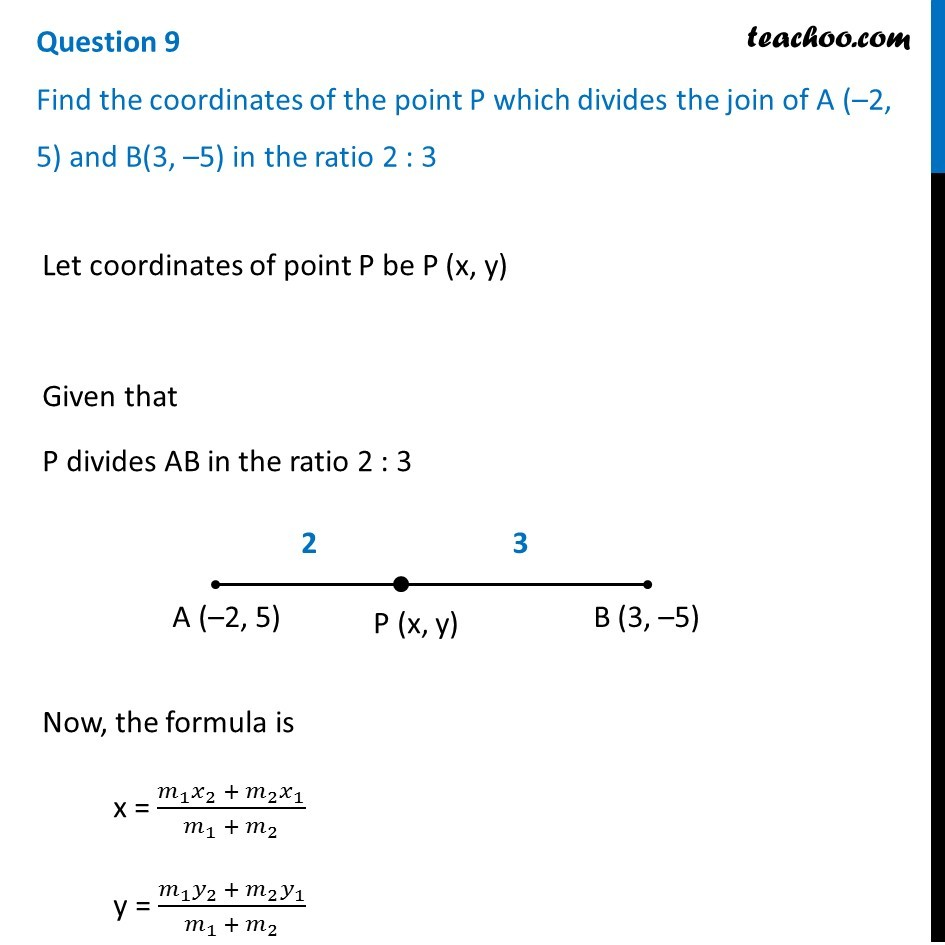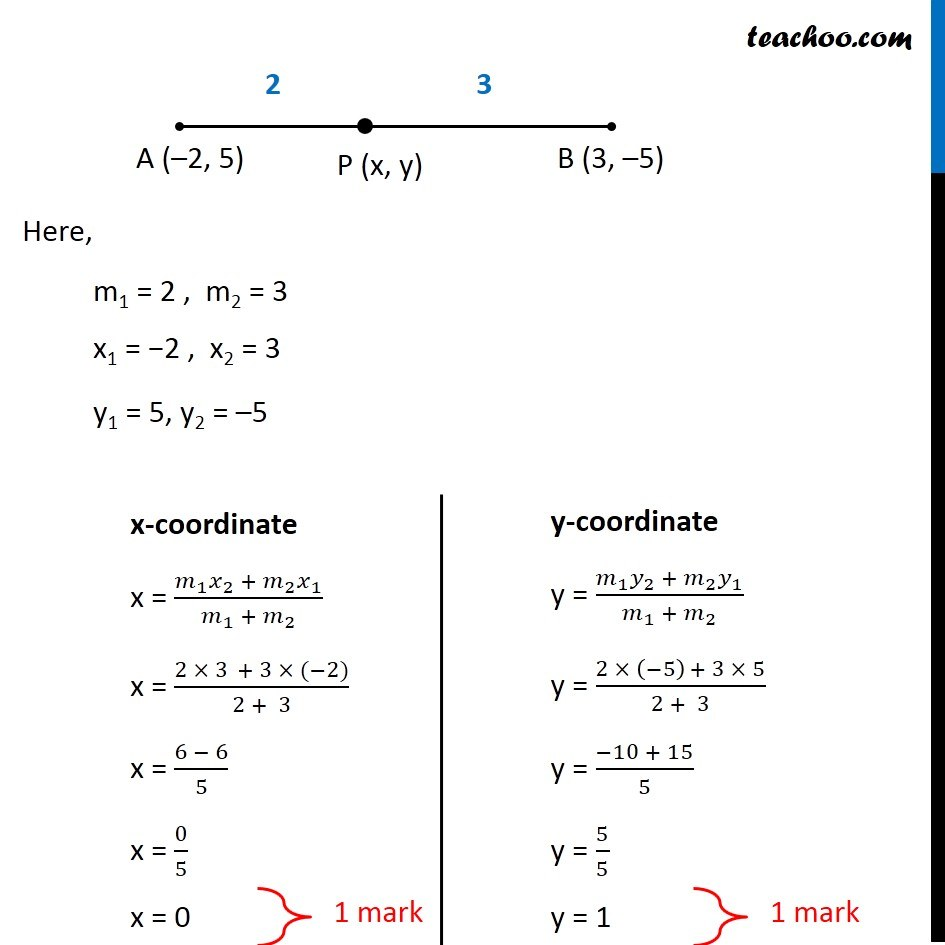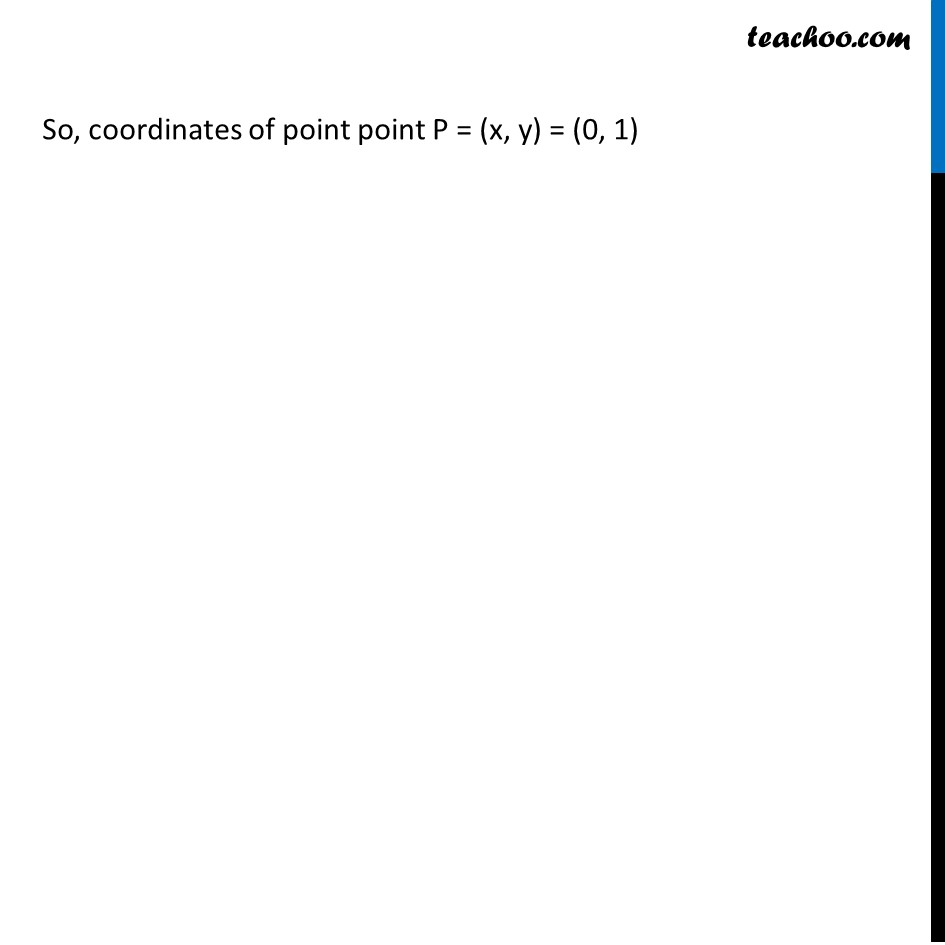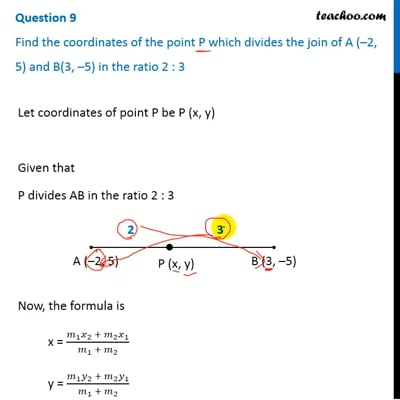CBSE Class 10 Sample Paper for 2019 Boards

Class 10
Solutions of Sample Papers for Class 10 Boards

Question 9

Find the coordinates of the point P which divides the join of A (–2, 5) and B(3, –5) in the ratio 2 : 3This video is only available for Teachoo black users

Introducing your new favourite teacher - Teachoo Black, at only ₹83 per month

### Transcript

Question 9 Find the coordinates of the point P which divides the join of A (–2, 5) and B(3, –5) in the ratio 2 : 3 Let coordinates of point P be P (x, y) Given that P divides AB in the ratio 2 : 3 Now, the formula is x = (𝑚_1 𝑥_2 + 𝑚_2 𝑥_1)/(𝑚_1 + 𝑚_2 ) y = (𝑚_1 𝑦_2 + 𝑚_2 𝑦_1)/(𝑚_1 + 𝑚_2 ) Here, m1 = 2 , m2 = 3 x1 = −2 , x2 = 3 y1 = 5, y2 = –5 x-coordinate x = (𝑚_1 𝑥_2 + 𝑚_2 𝑥_1)/(𝑚_1 + 𝑚_2 ) x = (2 × 3 + 3 × (−2))/(2 + 3) x = (6 − 6)/5 x = 0/5 x = 0 y-coordinate y = (𝑚_1 𝑦_2 + 𝑚_2 𝑦_1)/(𝑚_1 + 𝑚_2 ) y = (2 × (−5) + 3 × 5)/(2 + 3) y = (−10 + 15)/5 y = 5/5 y = 1 So, coordinates of point point P = (x, y) = (0, 1)